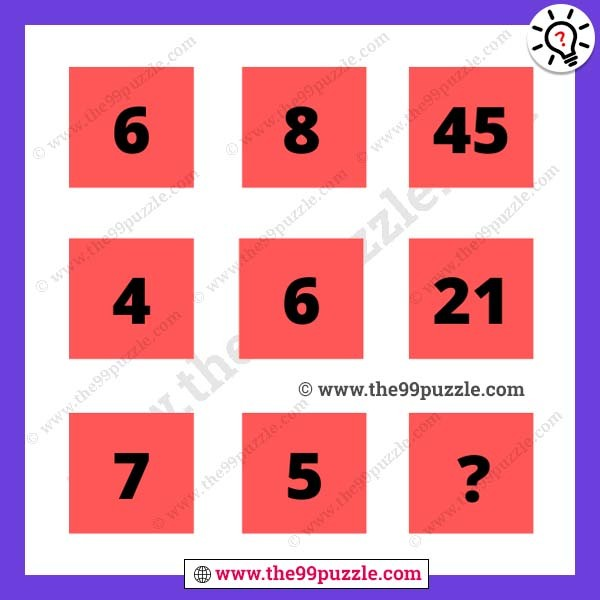Math reasoning for adults with the answer. This math logic question asks many competitive exams and interviews. Practice these puzzles that help you on exam time to quickly answer. Also, you improve your reasoning skill with these math puzzles. We are sharing many types of puzzles and riddles that are for all people. Various puzzle solving makes you smarter and more genius. Here this puzzle picture you see some numbers in the box in a logical format. You have to replace the question mark.###### Explanation:

1st row = (6-1)×(8+1) = 45

2nd row = (4-1)×(6+1) = 21

3rd row = (7-1)×(5+1) = 36